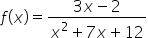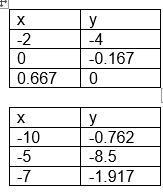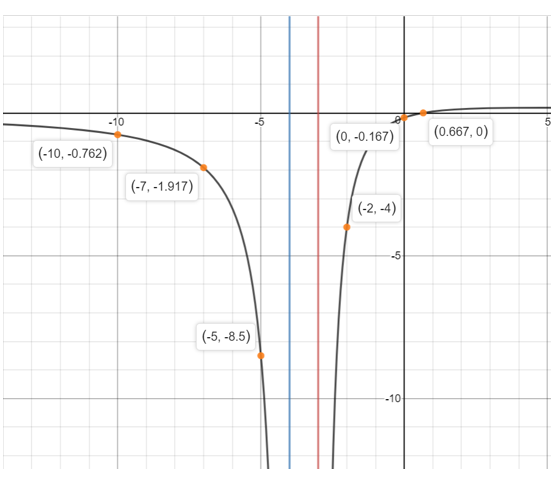Maths-
General
Easy

Question

# What are the horizontal asymptotes for the graphHint:

## The correct answer is: From the graph we can analyze that the vertical asymptote of the rational function is x = -3 and x = -4.

### Find the asymptotes of the rational function, if any. Draw the asymptotes as dotted lines. Find the x -intercept (s) and y -intercept of the rational function, if any. Find the values of y for several different values of x . Plot the points and draw a smooth curve to connect the points. Make sure that the graph does not cross the vertical asymptotes. The vertical asymptote of a rational function is x - value where the denominator of the function is zero. Equate the denominator to zero and find the value of x .x2 + 7x + 12 = 0x2 + 3x + 4x + 12 = 0x(x + 3) + 4(x + 3) = 0(x + 3) (x + 4) = 0x = -3   or   x = -4The vertical asymptote of the rational function is x =−3 and x = -4This function has x -intercept at (4,0) and y -intercept at (0,7) . We will find more points on the function and graph the function.From the graph we can analyze that the vertical asymptote of the rational function is  x = -3 and x = -4.#### With Turito Foundation.#### Get an Expert Advice From Turito.